# 5 ways to sum a column in Excel

This tutorial shows how to sum a column in Excel 2010 - 2016. Try out 5 different ways to total columns: find the sum of the selected cells on the Status bar, use AutoSum in Excel to sum all or only filtered cells, employ the SUM function or convert your range to Table for easy calculations.

If you store such data as price lists or expense sheets in Excel, you may need a quick way to sum up prices or amounts. Today I'll show you how to easily total columns in Excel. In this article, you'll find tips that work for summing up the entire column as well as hints allowing to sum only filtered cells in Excel.

Below you can see 5 different suggestions showing how to sum a column in Excel. You can do this with the help of the Excel SUM and AutoSum options, you can use Subtotal or turn your range of cells into Excel Table which will open new ways of processing your data.

### How to sum a column in Excel with one click

There is one really fast option. Just click on the letter of the column with the numbers you want to sum and look at the Excel Status bar to see the total of the selected cells.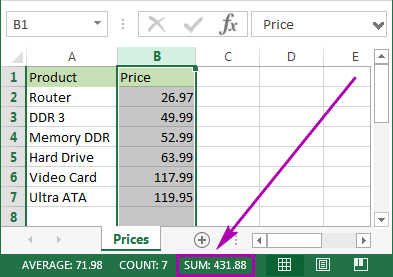Being really quick, this method neither allows copying nor displays numeric digits.

## How to total columns in Excel with AutoSum

If you want to sum up a column in Excel and keep the result in your table, you can employ the AutoSum function. It will automatically add up the numbers and will show the total in the cell you select.

1. To avoid any additional actions like range selection, click on the first empty cell below the column you need to sum.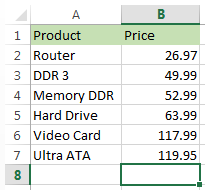2. Navigate to the Home tab -> Editing group and click on the AutoSum button.3. You will see Excel automatically add the =SUM function and pick the range with your numbers.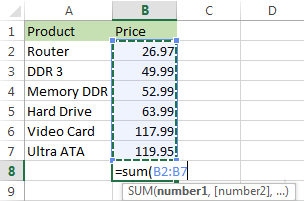4. Just press Enter on your keyboard to see the column totaled in Excel.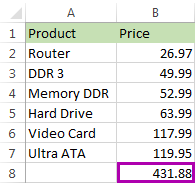This method is fast and lets you automatically get and keep the summing result in your table.

## Use the SUM function to total a column

You can also enter the SUM function manually. Why would you need this? To total only some of the cells in a column or to specify an address for a large range instead of selecting it manually.

1. Click on the cell in your table where you want to see the total of the selected cells.2. Enter `=sum(` to this selected cell.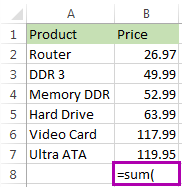3. Now select the range with the numbers you want to total and press Enter on your keyboard.

Tip. You can enter the range address manually like `=sum(B1:B2000)`. It's helpful if you have large ranges for calculation.That's it! You will see the column summed. The total will appear in the correct cell.This option is really handy if you have a large column to sum in Excel and don't want to highlight the range. However, you still need to enter the function manually. In addition, please be prepared that the SUM function will work even with the values from hidden and filtered rows. If you want to sum visible cells only, read on and learn how.

Tips:

## Use Subtotal in Excel to sum only filtered cells

This feature is perfect for totaling only the visible cells. As a rule, these are filtered or hidden cells.

1. First, filter your table. Click on any cell within your data, go to the Data tab and click on the Filter icon.2. You will see arrows appear in the column headers. Click on the arrow next to the correct header to narrow down the data.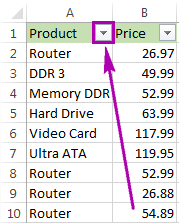3. Uncheck Select All and tick off only the value(s) to filter by. Click OK to see the results.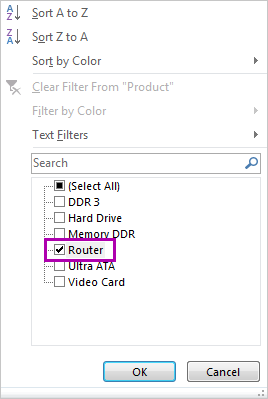4. Select the range with the numbers to add up and click AutoSum under the Home tab.Voila! Only the filtered cells in the column are summed up.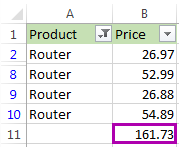If you want to sum visible cells but don't need the total to be pasted to your table, you can select the range and see the sum of the selected cells on the Excel Status bar. Or you can go ahead and see one more option for summing only filtered cells.

## Convert your data into Excel table to get total for your column

If you often need to sum columns, you can convert your spreadsheet to Excel Table. This will simplify totaling columns and rows as well as performing many other operations with your list.

1. Press Ctrl + T on yourkeyboardto format the range of cells as Excel Table.
2. You will see the new Design tab appear. Navigate to this tab and tick the checkbox Total Row.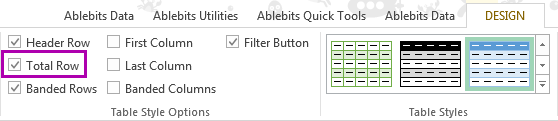3. A new row will be added at the end of your table. To make sure you get the sum, select the number in the new row and click on the small down arrow next to it. Pick the Sum option from the list.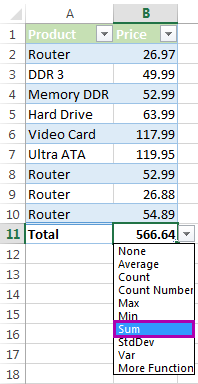Using this option lets you easily display totals for each column. You can see sum as well as many other functions like Average, Min and Max.

This feature adds up only visible (filtered) cells. If you need to calculate all data, feel free to employ instructions from How to total columns in Excel with AutoSum and Enter the SUM function manually to total the column.

Whether you need to sum the entire column in Excel or total only visible cells, in this article I covered all possible solutions. Choose an option that will work for your table: check the sum on the Excel Status bar, use the SUM or SUBTOTAL function, check out the AutoSum functionality or format your data as Table.

If you have any questions or difficulties, don't hesitate to leave comments. Be happy and excel in Excel!

## You may also be interested in

1. How do I add numbers in a column to get a total? I’ve tried highlighting the entire column, selecting auto sum and all I get is a formula =SUM(). In previous versions all I had to do was highlight the column I wanted to add select auto sum and then my total appeared in the empty cell below.

2. how about sum total formula from formula numbers how can i total, it cant be read if i used sum of..

• Hi!
What do you want to calculate exactly? Your question is not completely clear, please specify.

3. is there a way to assign a number value to a letter and do an auto sum from that?

4. Hi Ryan,

Thank you so much for a very helpful post. I am creating a timesheet template but the total for the hours worked is incorrect. On the particular cell, I used the formula: =SUM(G5:G11), and it should give the total number of hours worked in a week. Not sure what I am not doing right, the data entries are in the form: hhmmss.

5. Is there a way to sum a column where every cell is the sum of 2 different cells? Every time I try it adds the formulas together and I end up with my original number.
Ie
C3 =b2-b3
C4 =b3-b4
C5 =b4-b5
If c6 is my total how do I get c3+c4+c5?
Please forgive me I am extremely new to excel

• Hi!